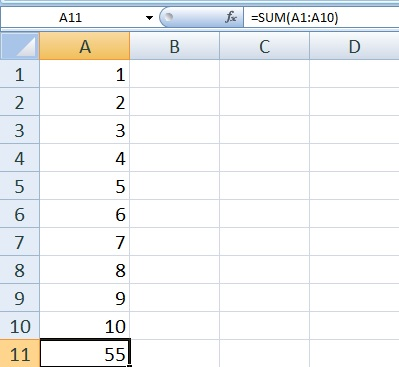The sum function is very useful in excel. It will give you the sum of the 1st cell to 255th cell.

Lets we see basic Sum Function

The sum is to add values. You can add two values and more than two values. As per the syntax you use.

=SUM (A1: A10)
=SUM (number1: number N)        Where N can be 2 to 255SUM Function =SUM(A1: A10)

This syntax will count the sum of the values contains in cell no A1 to A10. It counts only numerical values if you write a non-numerical then the system will count non-numerical as 0. It can sum up to the 1st cell to 255th cell.

=SUM (A1:A5,C6:C10)

=SUM (number N: number N, number N: number N)                                                                                                     Where N can be 1 to 255

This syntax will count the values of your selected cells. It is the flexible formula.

You just have to select first range and place comma and select the second range. That will give you the sum of both the selected ranges of your sheet.

### SUM at Status bar

If you select the data than status bar shows you SUM, AVERAGE and COUNTS of your data. It is one of the quickest ways to see SUM.

By using right click on the status bar will show other option. What you want the quick result of. Available options of status bar are SUM, AVERAGE, COUNT, NUMERICAL COUNT, MINIMUM, and MAXIMUM.

The flexible sum is used when you want to add different ranges of cells and neglect the specific or avoid the cells which you do no\t want to count in your sum.Sum Function Flexibility
Different ranges of the formula will highlight with different colors. It can calculate Row range with column range also.

### Using AutoSum

First of all you have to write your data and select the next cell from the last entry
Now Select Autosum option from the ribbon, Which is located at right upper corner of the workbook
or Use shortcut Key"Alt+="
Press enter

### Error Faced  in SUM Function

1. The sum value shows #### in place of the result
It means the return value is longer than the cell width. It is solved by expanding cell width

2.  In the result cell shows function but not the result value
if the values in the cell are formatted as text than this type of error comes. if you convert values to number from text this error will omit.

3.  Only numerical values are counted in sum. If non-numerical values are placed in the sum range system will count it as a 0.

4.  #NAME
This is a syntax error. If you have placed or by mistake an extra character existed in formula than this type of error occur. For Ex =SUM(A1:\A10)